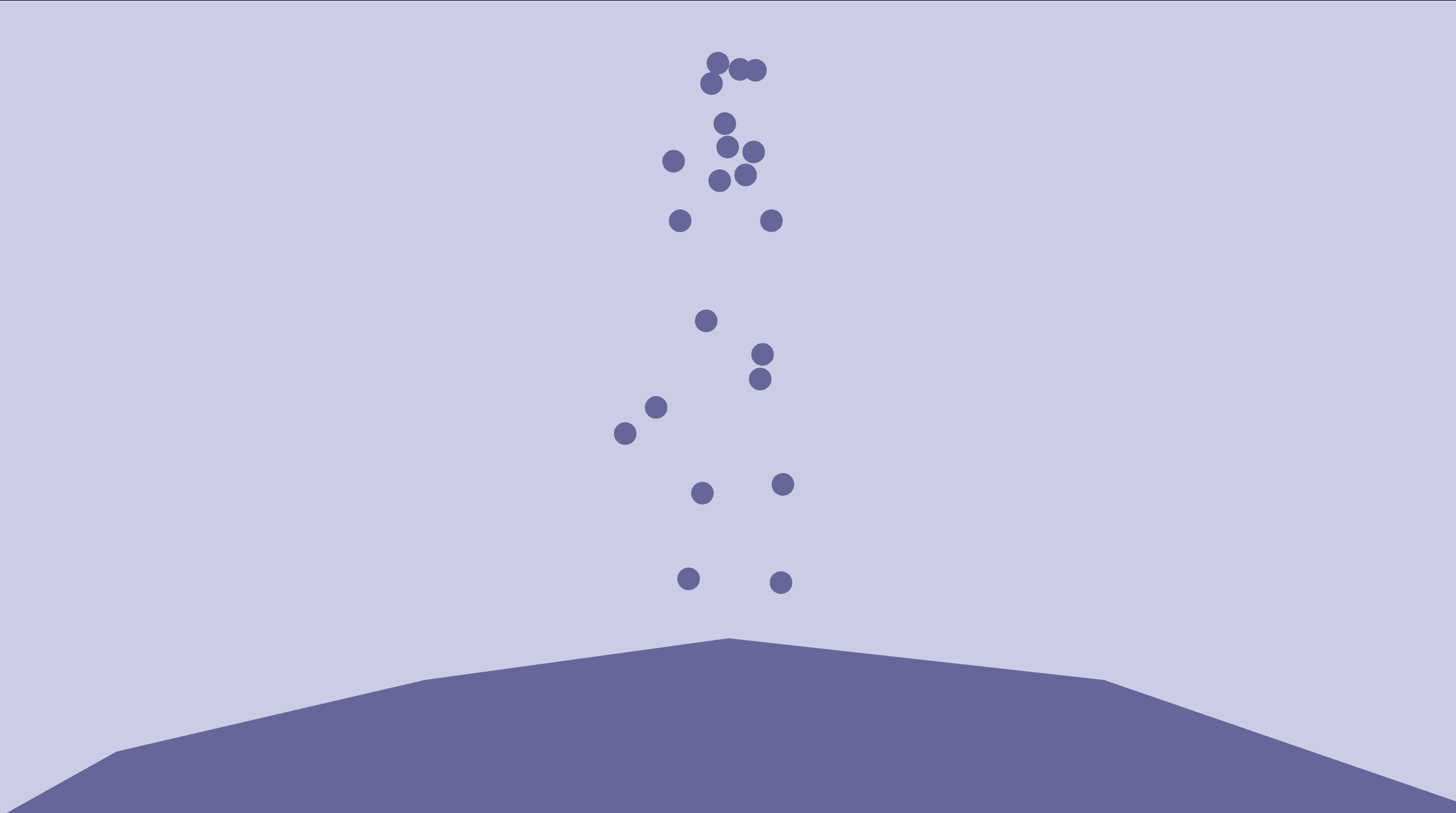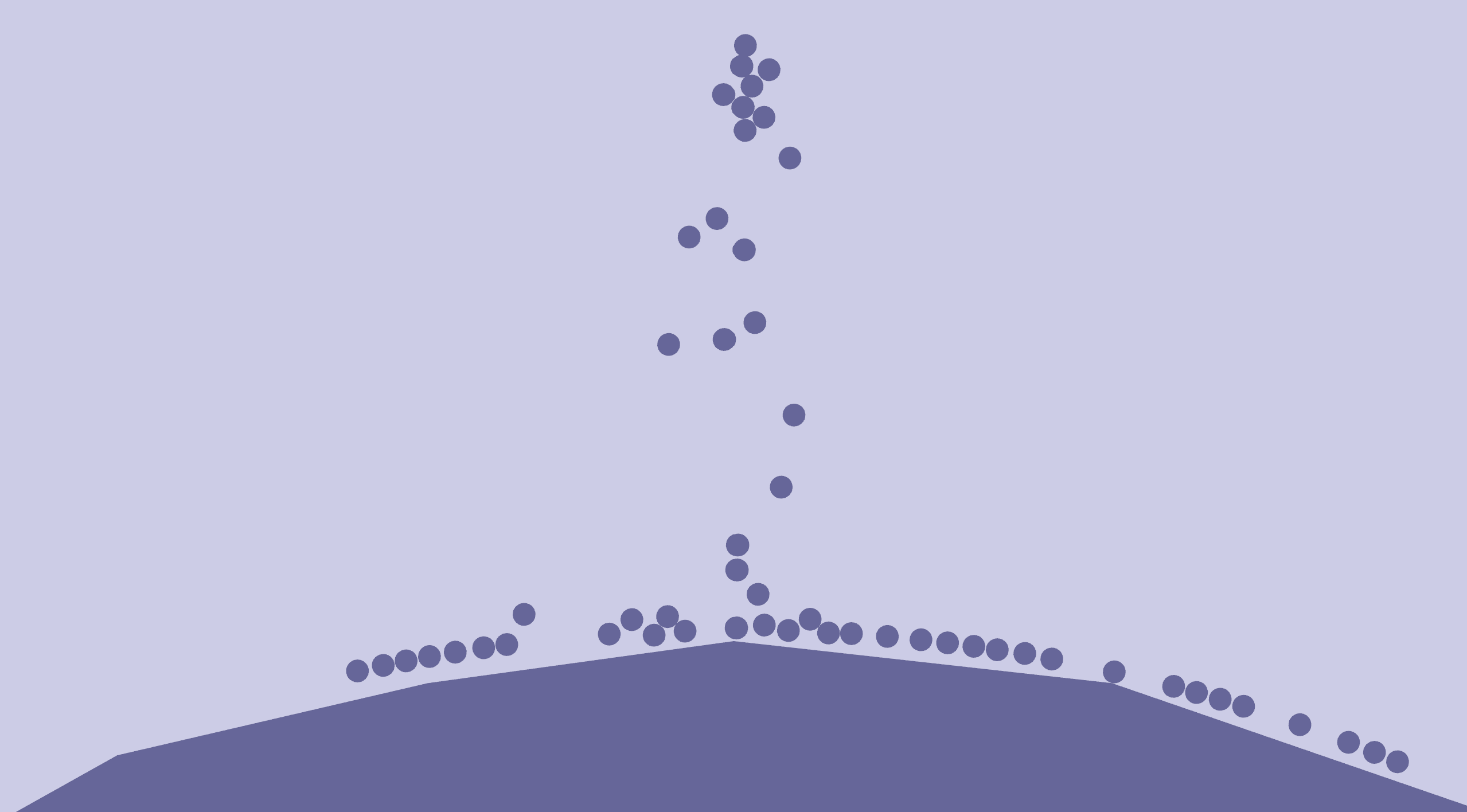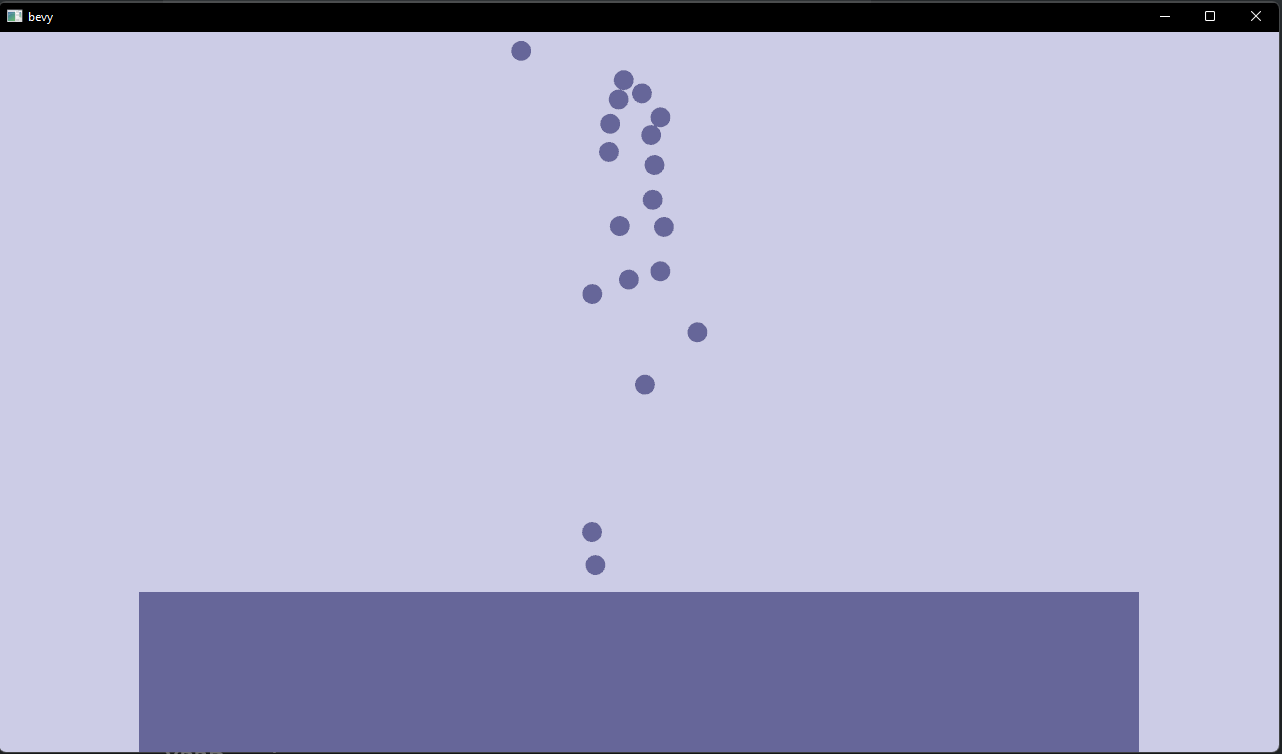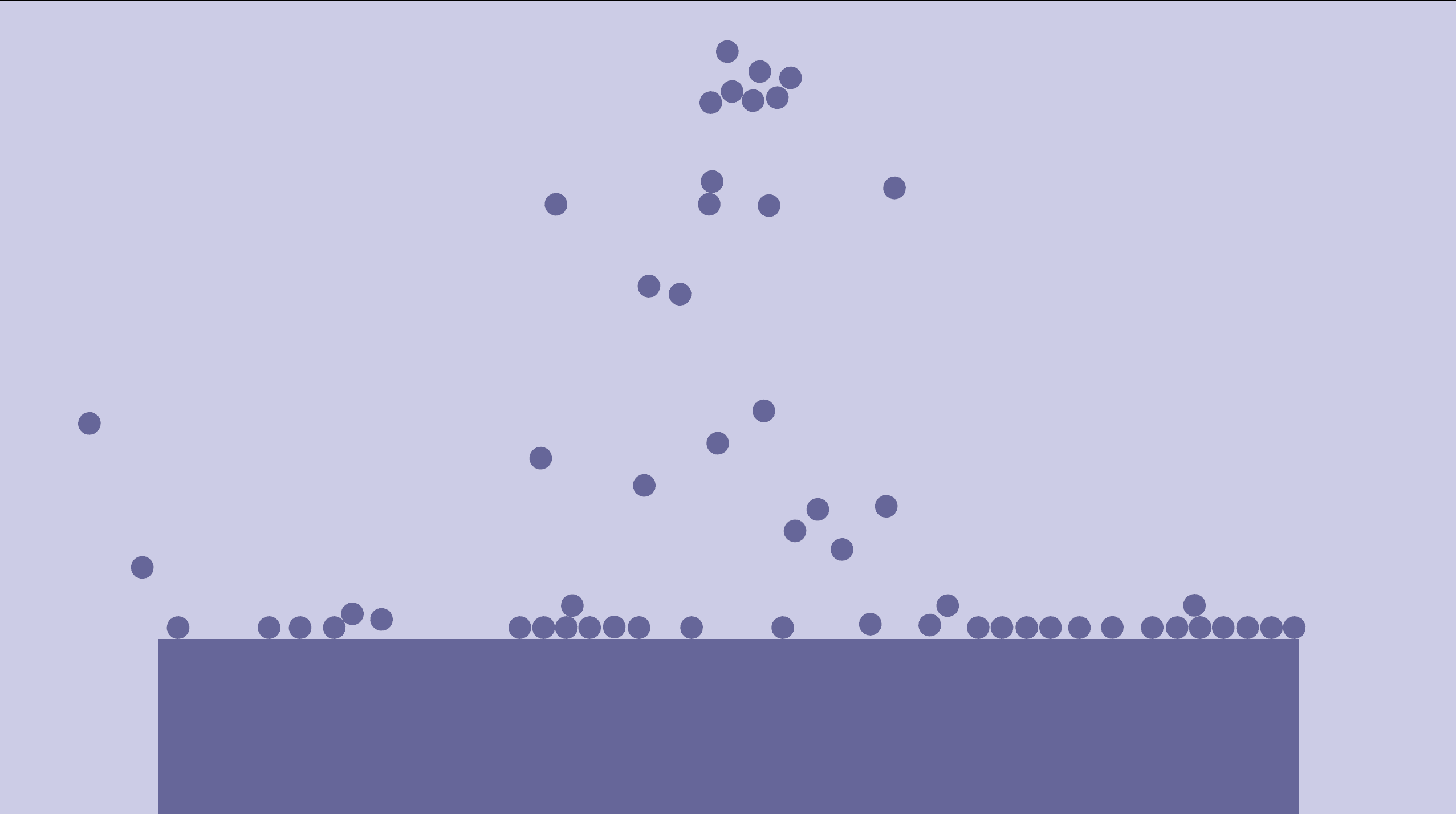# Physics engine - Part 2: Static boxes

In this part, we'll add support for static colliders, including boxes.

## A more interesting example

So if you're a bit bored with all the two-particle examples already, I've got some good news for you! Next we will be adding an example where we pour marbles onto a floor.

Create a new example, marble_pour.rs and paste in the following:

use bevy::{core::FixedTimestep, prelude::*};
use bevy_xpbd::*;
use rand::random;

fn main() {
App::new()
.insert_resource(ClearColor(Color::rgb(0.8, 0.8, 0.9)))
.insert_resource(Msaa { samples: 4 })
SystemSet::new()
.with_run_criteria(FixedTimestep::step(1. / 20.))
.with_system(spawn_marble),
)
.run();
}

struct Materials {
blue: Handle<StandardMaterial>,
}

struct Meshes {
sphere: Handle<Mesh>,
}

fn startup(
mut commands: Commands,
mut meshes: ResMut<Assets<Mesh>>,
mut materials: ResMut<Assets<StandardMaterial>>,
) {
commands.insert_resource(Meshes {
subdivisions: 4,
})),
});

commands.insert_resource(Materials {
base_color: Color::rgb(0.4, 0.4, 0.6),
unlit: true,
..Default::default()
}),
});

commands.spawn_bundle(OrthographicCameraBundle {
transform: Transform::from_translation(Vec3::new(0., 0., 100.)),
orthographic_projection: bevy::render::camera::OrthographicProjection {
scale: 0.01,
..Default::default()
},
..OrthographicCameraBundle::new_3d()
});
}

fn spawn_marbles(mut commands: Commands, materials: Res<Materials>, meshes: Res<Meshes>) {
let pos = Vec2::new(random::<f32>() - 0.5, random::<f32>() - 0.5) * 0.5 + Vec2::Y * 3.;
let vel = Vec2::new(random::<f32>() - 0.5, random::<f32>() - 0.5);
commands
.spawn_bundle(PbrBundle {
mesh: meshes.sphere.clone(),
material: materials.blue.clone(),
transform: Transform {
translation: pos.extend(0.),
..Default::default()
},
..Default::default()
})
.insert_bundle(ParticleBundle {
..ParticleBundle::new_with_pos_and_vel(pos, vel)
});
}

fn despawn_marbles(mut commands: Commands, query: Query<(Entity, &Pos)>) {
for (entity, pos) in query.iter() {
if pos.0.y < -20. {
commands.entity(entity).despawn();
}
}
}


It uses the rand crate to add some randomness, so add rand to your dev-dependencies in Cargo.toml:

[dev-dependencies]
rand = "0.8"


Running it you should see a nice pour of blue particles falling down.Having some marbles falling down is cool, but wouldn't it be more interesting if the marbles could land on the a floor? Now, the question is, how do we add a floor? So far all we have are circular particles, but the floor should be flat, and it shouldn't be affected by gravity or moved by the particles.

Let's handle the last two points first, and look into making it flat afterwards. What we want to add, is called static colliders. That is we want to add entities that have a position and a shape, but do not move.

We will approximate the floor by adding a really big static circle below the flow of marbles. We can't use our ParticleBundle for this, as that includes the Vel, PrevPos and PreSolveVel components, which do not makes sense for things that do no move (or at the very least it's a waste of memory). So let's create a new StaticColliderBundlethat has those components removed. That leaves us with Pos , CircleCollider, Restitution and Mass. Mass for statics is a bit weird... In one way statics can be seen as having infinite mass (remember penetration constraints are enforced according to their inverse mass, which would mean statics would have an inverse mass of 0 and wouldn't be affected at all). We could also just remove the Mass component and have a special system for handling statics. We will do the latter. Restitution looks like it doesn't make sense either, since statics won't bounce, but remember that when two objects collide we take the average of their restitutions, so it does make sense to keep it, so we can create bouncy and non-bouncy floors for instance.

That leaves us with the following bundle:

#[derive(Bundle, Default)]
pub struct StaticColliderBundle {
pub pos: Pos,
pub collider: CircleCollider,
pub restitution: Restitution,
}


Let's use our shiny new component to add a nice big static circle to our example:

// fn startup()

subdivisions: 4,
}));

base_color: Color::rgb(0.4, 0.4, 0.6),
unlit: true,
..Default::default()
});

commands
.spawn_bundle(PbrBundle {
mesh: sphere.clone(),
material: blue.clone(),
transform: Transform {
..Default::default()
},
..Default::default()
})
.insert_bundle(StaticColliderBundle {
..Default::default()
});

commands.insert_resource(Meshes { sphere });
commands.insert_resource(Materials { blue });


To be honest, it doesn't actually look that nice. If you run the example, you will see that it's quite jagged and a crude approximation of a circle as we don't have that many subdivisions in our sphere mesh, but let's just pretend it looks nice and circular. We will replace it soon anyway.The other thing you'll notice, is that nothing is actually colliding with it... The particles just go straight through it. Let's fix that!

The reason it's not working, is that our solve_pos and solve_vel systems have queries that require a bunch of the components that we just removed.

The most straightforward way to solve this, is to add new systems for handling collisions between static objects and dynamics (things that move). Let's add a new solve_pos_statics system:

fn solve_pos_statics(
mut dynamics: Query<(&mut Pos, &CircleCollider), With<Mass>>,
statics: Query<(&Pos, &CircleCollider), Without<Mass>>,
) {
for (mut pos_a, circle_a) in dynamics.iter_mut() {
for (pos_b, circle_b) in statics.iter() {
let ab = pos_b.0 - pos_a.0;
let ab_sqr_len = ab.length_squared();
let ab_length = ab_sqr_len.sqrt();
let penetration_depth = combined_radius - ab_length;
let n = ab / ab_length;
pos_a.0 -= n * penetration_depth;
}
}
}
}


This should somewhat familiar to you. In a way it's a copy of the solve_pos system, but it turns out a little simpler. First, since we have two disjoint queries (we distinguish between them using the With/ Without Mass filters), we can have a simple nested loop instead of using iter_combinations(). We can also remove the masses entirely from the equations, as the static object having infinite mass means the net result is that we will only move the dynamic object.

Let's register our new system below the solve_pos system:

                .with_system(
solve_pos
.label(Step::SolvePositions)
.after(Step::Integrate),
)
.with_system(
solve_pos_statics
.label(Step::SolvePositions)
.after(Step::Integrate),
)


We now have two systems that have the same label and the same and same ordering requirements. This totally makes sense, as they do correspond to the same SolvePositions step in the XPBD algorithm. Systems in bevy are allowed to share the same level. And here it doesn't matter a lot in which order the two solve systems are run, just that they run after the Integrate step and before the UpdateVelocities step, and this is ensured here. We can, however, rewrite this to get rid of some of the duplication, and better communicate our intent:

                .with_system_set(
SystemSet::new()
.label(Step::SolvePositions)
.after(Step::Integrate)
.with_system(solve_pos)
.with_system(solve_pos_statics),
)


This is equivalent to what we had before, and adds the same label to both systems.

If you run the example now, the balls should have stopped falling through the floor. They don't bounce yet, however.

So, to solve that, we once again need to implement the SolveVelocities step for this new group of entities. That means: create a solve_vel_statics, simplify the parts where velocities are known to always be 0 and where the mass is known to be infinite.

However, there is one thing we need to do first. We want to iterate over contact pairs, but the Contacts resource currently only stores contacts between dynamic objects. We could begin adding contacts between static and dynamic objects to it as well (in solve_pos_statics), however, then we would have to check as we iterate over contact pairs in solve_vel (which handles dynamic contacts) to make sure we don't accidentally try to unwrap the wrong type of entity pair. So instead, we create yet another resource, StaticContacts:

#[derive(Default, Debug)]
pub struct StaticContacts(pub Vec<(Entity, Entity)>);

// ...

app.init_resource::<Gravity>()
.init_resource::<Contacts>()
.init_resource::<StaticContacts>()


And then we need to maintain it in solve_pos_statics:

fn solve_pos_statics(
mut dynamics: Query<(Entity, &mut Pos, &CircleCollider), With<Mass>>, // <-- new
statics: Query<(Entity, &Pos, &CircleCollider), Without<Mass>>, // <-- new
mut contacts: ResMut<StaticContacts>, // <-- new
) {
contacts.0.clear(); // <-- new
for (entity_a, mut pos_a, circle_a) in dynamics.iter_mut() {
for (entity_b, pos_b, circle_b) in statics.iter() {
let ab = pos_b.0 - pos_a.0;
let ab_sqr_len = ab.length_squared();
let ab_length = ab_sqr_len.sqrt();
let penetration_depth = combined_radius - ab_length;
let n = ab / ab_length;
pos_a.0 -= n * penetration_depth;
contacts.0.push((entity_a, entity_b)); // <-- new
}
}
}
}


And finally, we can implement solve_vel_statics:

fn solve_vel_statics(
mut dynamics: Query<(&mut Vel, &PreSolveVel, &Pos, &Restitution), With<Mass>>,
statics: Query<(&Pos, &Restitution), Without<Mass>>,
contacts: Res<StaticContacts>,
) {
for (entity_a, entity_b) in contacts.0.iter().cloned() {
let (mut vel_a, pre_solve_vel_a, pos_a, restitution_a) =
dynamics.get_mut(entity_a).unwrap();
let (pos_b, restitution_b) = statics.get(entity_b).unwrap();
let ba = pos_a.0 - pos_b.0;
let n = ba.normalize();
let pre_solve_normal_vel = Vec2::dot(pre_solve_vel_a.0, n);
let normal_vel = Vec2::dot(vel_a.0, n);
let restitution = (restitution_a.0 + restitution_b.0) / 2.;
vel_a.0 += n * (-normal_vel - restitution * pre_solve_normal_vel);
}
}


Once again, static collisions are a lot simpler than the dynamic ones. Let's also register our new system using the same pattern as for the position solve. Replace the existing solve_vel registration with the following:

               .with_system_set(
SystemSet::new()
.label(Step::SolveVelocities)
.after(Step::UpdateVelocities)
.with_system(solve_vel)
.with_system(solve_vel_statics),
)


If you run the example now, should see the marbles being pushed out and often bounce off of our static circle.Phew, let's take a break and enjoy the shower of circles.

Now before we wrap up this section, there is one thing we should fix first: We are computing the normals twice, once when solving positions, and again when solving velocities.

In a way, it's totally fine for now, but as we add more types of shapes, it will start to make sense. Adding the normals to the contact pairs allow us to remove the circle-specific computation from solve_vel and solve_vel_statics, making it completely decoupled from what kind of shapes collided. Doing this now saves us a lot of work down the line. Add new normal fields to the *Contacts resource tuples:

#[derive(Default, Debug)]
pub struct Contacts(pub Vec<(Entity, Entity, Vec2)>);

#[derive(Default, Debug)]
pub struct StaticContacts(pub Vec<(Entity, Entity, Vec2)>);


And update the solve_pos* systems to store them:

// fn solve_pos()
contacts.0.push((entity_a, entity_b, n));
// fn solve_pos_statics()
contacts.0.push((entity_a, entity_b, n));


And use them in the solve_vel* systems:

fn solve_vel(
mut query: Query<(&mut Vel, &PreSolveVel, &Mass, &Restitution)>,
contacts: Res<Contacts>,
) {
for (entity_a, entity_b, n) in contacts.0.iter().cloned() {
let (
(mut vel_a, pre_solve_vel_a, mass_a, restitution_a),
(mut vel_b, pre_solve_vel_b, mass_b, restitution_b),
) = unsafe {
// Ensure safety
assert!(entity_a != entity_b);
(
query.get_unchecked(entity_a).unwrap(),
query.get_unchecked(entity_b).unwrap(),
)
};
let pre_solve_relative_vel = pre_solve_vel_a.0 - pre_solve_vel_b.0;
let pre_solve_normal_vel = Vec2::dot(pre_solve_relative_vel, n);

let relative_vel = vel_a.0 - vel_b.0;
let normal_vel = Vec2::dot(relative_vel, n);
let restitution = (restitution_a.0 + restitution_b.0) / 2.;

let w_a = 1. / mass_a.0;
let w_b = 1. / mass_b.0;
let w_sum = w_a + w_b;

let restitution_velocity = (-restitution * pre_solve_normal_vel).min(0.);
let vel_impulse = n * ((-normal_vel + restitution_velocity) / w_sum);

vel_a.0 += vel_impulse * w_a;
vel_b.0 -= vel_impulse * w_b;
}
}

fn solve_vel_statics(
mut dynamics: Query<(&mut Vel, &PreSolveVel, &Restitution), With<Mass>>,
statics: Query<&Restitution, Without<Mass>>,
contacts: Res<StaticContacts>,
) {
for (entity_a, entity_b, n) in contacts.0.iter().cloned() {
let (mut vel_a, pre_solve_vel_a, restitution_a) = dynamics.get_mut(entity_a).unwrap();
let restitution_b = statics.get(entity_b).unwrap();
let pre_solve_normal_vel = Vec2::dot(pre_solve_vel_a.0, n);
let normal_vel = Vec2::dot(vel_a.0, n);
let restitution = (restitution_a.0 + restitution_b.0) / 2.;
vel_a.0 += n * (-normal_vel + (-restitution * pre_solve_normal_vel).min(0.));
}
}


Notice how we could now also remove the Pos components from our queries.

It's time to fix that rough floor and add some basic support for boxes!

Let's add a new type of collider component BoxCollider:

// components.rs

#[derive(Component, Debug)]
pub struct BoxCollider {
pub size: Vec2,
}

impl Default for BoxCollider {
fn default() -> Self {
Self { size: Vec2::ONE }
}
}


But wait! Usually, we would head over entities.rs and add the new component to our bundle, but this time we got ourselves in sort of a situation. Our bundles already have a collider field defined, and it's occupied by a CircleCollider component. So how do we get around it?

One (very tempting) option would be to make a Collider enum that could either be a Circle with a radius or a Box with width and height.

This would mean we would have to match on the Collider enum deep inside all of our solve_* systems. It certainly would work, however, there is something really nice to be said about being able avoid that kind of branching at one of the most performance-critical parts of our code. And it's really nice to be able to write queries that go through all circles, or all boxes. We'd lose that if we hide the details away in an enum component.

So let's try to stick with separate components for now and see where it leads us!

We'll keep it simple-stupid at first, and we just create a new bundle for boxes. We'll start with the static case and rename the existing StaticColliderBundle to StaticCircleBundle and create a new StaticBoxBundle:

#[derive(Bundle, Default)]
pub struct StaticCircleBundle {
pub pos: Pos,
pub collider: CircleCollider,
pub restitution: Restitution,
}

#[derive(Bundle, Default)]
pub struct StaticBoxBundle {
pub pos: Pos,
pub collider: BoxCollider,
pub restitution: Restitution,
}


Now there will be some repetition, which might rub you the wrong way, but we'll get back to it. Promise!

Now that we have the components in place, let's use it to create flat floor in the marble_pour example. Instead of the StaticColliderBundle lets use our new StaticBoxBundle instead:

    let size = Vec2::new(10., 2.);
commands
.spawn_bundle(PbrBundle {
material: blue.clone(),
transform: Transform::from_scale(size.extend(1.)),
..Default::default()
})
.insert_bundle(StaticBoxBundle {
pos: Pos(Vec2::new(0., -3.)),
collider: BoxCollider { size },
..Default::default()
});


Your example should now look something like this:That's more like it! But the circles pass through the ground again. To fix it we need to implement systems that handle collisions for boxes. solve_pos_statics is already shape agnostic, so that's fine (see, I said it'll pay off), but we still need to solve position constraints between dynamic spheres and static boxes and also add the collisions to the StaticContacts resource.

StaticContacts is currently being cleared inside solve_pos_statics , so we need to sure that clearing is not happening after we've added our boxes. We could solve this by simply ordering our new system after solve_pos, but let's create a new dedicated clear_contacts system instead, and also clear the dynamic-dynamic contacts (Contacts).

fn clear_contacts(mut contacts: ResMut<Contacts>, mut static_contacts: ResMut<StaticContacts>) {
contacts.0.clear();
static_contacts.0.clear();
}


And make sure to remove the contacts.0.clear() call from solve_pos and solve_pos_statics

Now add new system to the schedule before the SolvePositions step:

                    .with_system(integrate.label(Step::Integrate))
.with_system(clear_contacts.before(Step::SolvePositions)) // <-- new
.with_system_set(
SystemSet::new()
.label(Step::SolvePositions)


Finally, we're ready to implement the new dynamic circle/static box collision system:

fn solve_pos_static_boxes(
mut dynamics: Query<(&mut Pos, &CircleCollider), With<Mass>>,
statics: Query<(&Pos, &BoxCollider), Without<Mass>>,
mut contacts: ResMut<StaticContacts>,
) {
for (mut pos_a, circle_a) in dynamics.iter_mut() {
for (pos_b, box_b) in statics.iter() {
// TODO: if colliding, move so not colliding, update contacts
}
}
}


We're checking for collision between a circle and a box. Since we haven't added rotations yet, we know that the box will be axis aligned, so the check isn't too complicated. We exploit the symmetry by taking the absolute value of the vector from the box center to the circle center. Now we only have to deal with the first quadrant. Then we check if the point is completely outside the rectangle. And then we handle the three cases (corner, closest to x edge and closest to y edge):

fn solve_pos_static_boxes(
mut dynamics: Query<(Entity, &mut Pos, &CircleCollider), With<Mass>>,
statics: Query<(Entity, &Pos, &BoxCollider), Without<Mass>>,
mut contacts: ResMut<StaticContacts>,
) {
for (entity_a, mut pos_a, circle_a) in dynamics.iter_mut() {
for (entity_b, pos_b, box_b) in statics.iter() {
let box_to_circle = pos_a.0 - pos_b.0;
let box_to_circle_abs = box_to_circle.abs();
let half_extents = box_b.size / 2.;
let corner_to_center = box_to_circle_abs - half_extents;
if corner_to_center.x > r || corner_to_center.y > r {
continue;
}

let s = box_to_circle.signum();

let (n, penetration_depth) = if corner_to_center.x > 0. && corner_to_center.y > 0. {
// Corner case
let corner_to_center_sqr = corner_to_center.length_squared();
if corner_to_center_sqr > r * r {
continue;
}
let corner_dist = corner_to_center_sqr.sqrt();
let penetration_depth = r - corner_dist;
let n = corner_to_center / corner_dist * -s;
(n, penetration_depth)
} else if corner_to_center.x > corner_to_center.y {
// Closer to vertical edge
(Vec2::X * -s.x, -corner_to_center.x + r)
} else {
(Vec2::Y * -s.y, -corner_to_center.y + r)
};

pos_a.0 -= n * penetration_depth;
contacts.0.push((entity_a, entity_b, n));
}
}
}


And add it to the SolvePositions step:

                    SystemSet::new()
.label(Step::SolvePositions)
.after(Step::Integrate)
.with_system(solve_pos)
.with_system(solve_pos_statics)
.with_system(solve_pos_static_boxes),


That's it, the balls should stop going through the floor, and since we add them to the list of static contacts, the velocity solve is handled the same way as for the circles (in solve_vel_statics).

If you run the example now, the falling marbles should bounce slightly when they hit the floor.So we could go ahead and add solver systems for dynamic rectangles as well. However that would require us to write at least four systems:

1. solve_pos_circle_box
2. solve_pos_box_box
3. solve_pos_static_circle_box
4. solve_pos_static_box_box

None of them are hard to write, but I want to get on with the rest of the XPBD algorithm and also add rotation first. So let's put a pin in it for now.